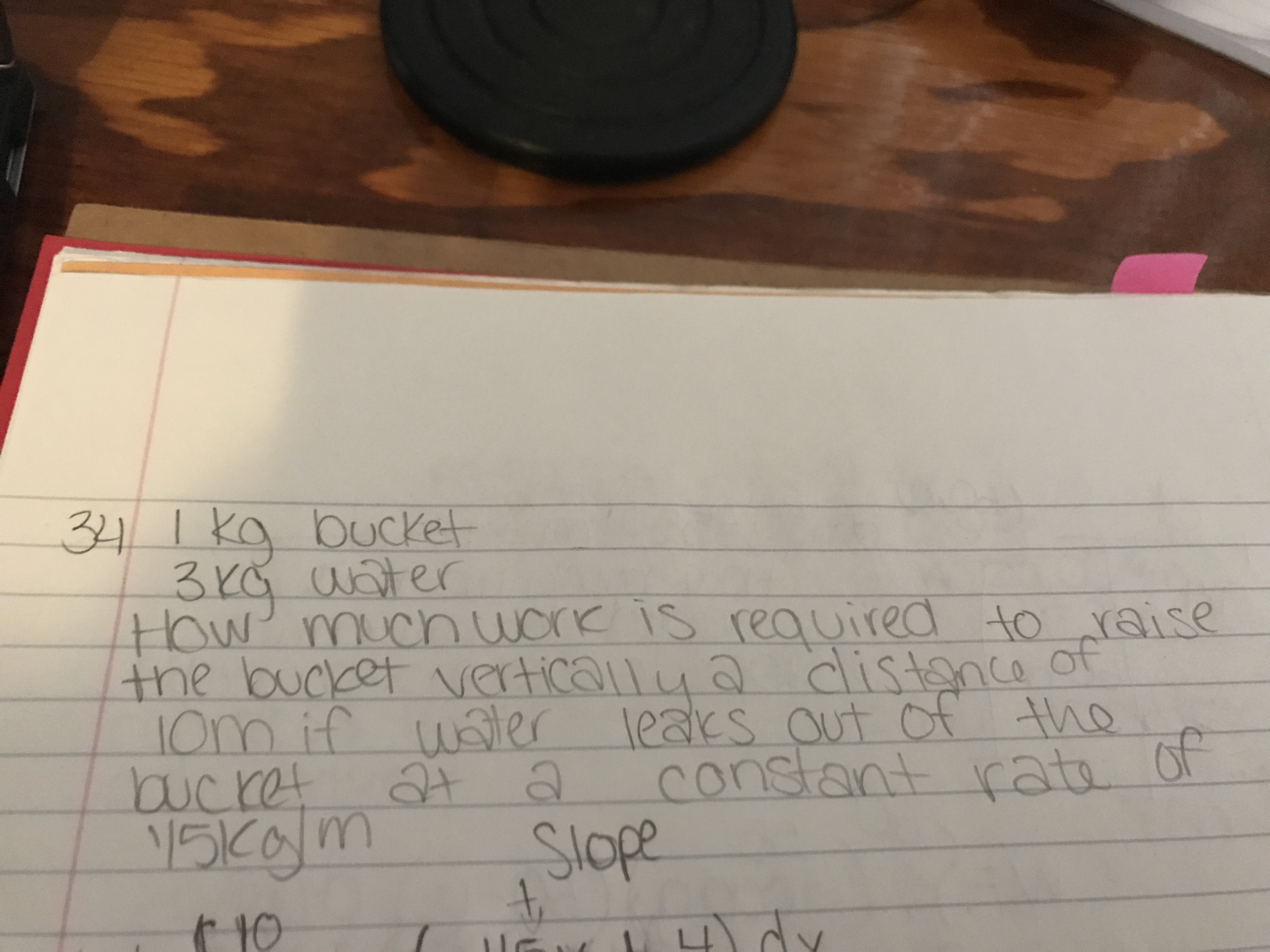Question
1 viewshelp_outlineImage Transcriptioncloseuoter ow' mucn worK iS reauired to raise Slope fullscreen
check_circle

Step 1

Initial mass, M0 = Mass of the bucket + mass of the water = 1 + 3 = 4 kg

Water leaks at a constant rate, k = 1/5 kg / m = 0.2 kg / m

Step 2

Let's say at some point "x" meters away from the ground, the remaining mass, M will be = Intial mass - leakage rate x distance away from the ground

Hence, M = M0 - kx

Step 3

So, gravitational force acting on the bucket F = Mg = (M0 -kx)g where g = accelration due to gravity = 9.8 m / s2

Hence, work...

### Want to see the full answer?

See Solution

#### Want to see this answer and more?

Solutions are written by subject experts who are available 24/7. Questions are typically answered within 1 hour.*

See Solution
*Response times may vary by subject and question.
Tagged in

### Calculus